# New Pattern Quantitative Aptitude Questions Based on IBPS PO Mains 2018 (Day-04)

Dear Aspirants, Quantitative Aptitude plays a crucial role in Banking and all other competitive exams. To enrich your preparation, here we have provided New Pattern Aptitude Questions that are similar to the questions asked in IBPS PO Mains 2018. Candidates those who are going to appear in upcoming bank exams like IBPS Clerk, IBPS SO, etc., can practice these questions daily and make your preparation effective.

[WpProQuiz 4543]

Directions (Q. 1 – 5): The questions below are based on the given Series-I. The series-I satisfy a certain pattern, follow the same pattern in Series-II and answer the questions given below.

1)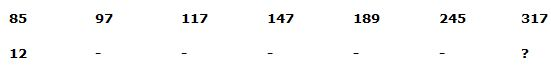a) 145

b) 278

c) 244

d) 176

e) 132

2)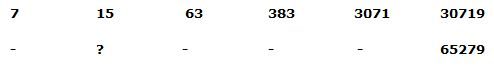a) 33

b) 21

c) 39

d) 27

e) 42

3)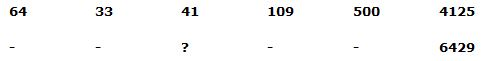a) 56

b) 62

c) 84

d) 77

e) 68

4)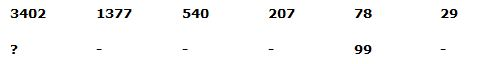a) 4852

b) 5103

c) 5376

d) 4527

e) 3964

5)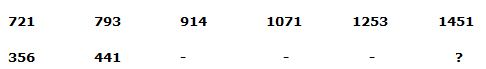a) 1258

b) 1363

c) 1151

d) 1076

e) 995

Directions (6-10): Study the following information carefully and answer the given questions.

The bar graph shows the percentage of profit earned by two different companies in different years.

The table shows the expenditure of company A and B (in lakhs)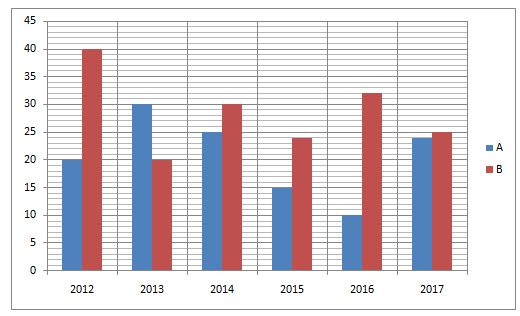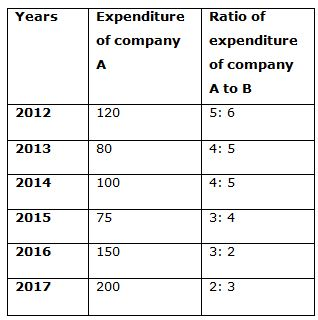6) Quantity I: In 2018, total income of company A and B is 500 lakhs and the expenditure of both companies is equal. Profit of company B is 20 lakhs more than the profit of company A and the profit percentage of company A is 20%. Company A’s profit is what percentage less than the company B’s profit?

Quantity II: Total income of company A and B in the year 2014 is approximately what percentage more/less than the total income of company A and B together in the year 2016?

Quantity III: Total expenditure of company B in the year 2013 and 2014 together is what percentage more/less than the total expenditure of company A in the year 2012 and 2014 together?

Which of the following should be placed in the blank spaces of the expression Quantity I__ Quantity II___Quantity III from left to right with respect to the above statements?

a) <, <

b) <, >

c) >, >

d) =, <

e) >, =

7) Quantity I: Find the difference of total income of company A in the year 2015, 2016 and 2017 together to that of total expenditure of company B in the year 2012, 2013 and 2014 together?

Quantity II: What is the average income of company B in the year 2014 and 2015 together?

Quantity III: In 2012, total expenditure of company A is 20% more than the previous year. The income of company A in the year 2013 is 20% less than the income of company A in the year 2011. Find the profit of company A in the year 2011?

Which of the following should be placed in the blank spaces of the expression Quantity I __ Quantity II ___ Quantity III from left to right with respect to the above statements?

a) =, <

b) <, >

c) >, =

d) =, =

e) ≥, >

8) Quantity I: In 2015, If the expenditure of company A is decreased by 25% and the income of company A is increased by 30%. Find the new profit percentage of company A?

Quantity II: In 2016, If the income of company B is decreased by 20% and the profit is decreased by (x) %. Find the value of x, if the expenditure is same.

Quantity III: Total income of company B in the year 2013 is what percentage more/less than the total expenditure of company A in the same year?

Which of the following should be placed in the blank spaces of the expression Quantity I __ Quantity II ___ Quantity III from left to right with respect to the above statements?

a) <, <

b) <, =

c) ≤, <

d) =, <

e) >, >

9) Quantity I: In Company A, expenditure in the year 2012, 2014 and 2016 is increased by x %, (x+10) % and (x+15) % respectively. Find the new income of company A in the year 2012, 2014 and 2016 together, if the total expenditure of company A in the year 2012, 2014 and 2016 together after increment is 439.5 lakhs and the profit percentage is same as previous?

Quantity II: In Company B, the income in the year 2013, 2015 and 2017 is decreased by 20%, 24% and 32% respectively. Find the new expenditure in the year 2013, 2015 and 2017 together if the profit percentage is same as previous?

Quantity III: Find the total income of company B in the year 2014, 2015 and 2016 together?

Which of the following should be placed in the blank spaces of the expression Quantity I__ Quantity II ___ Quantity III from left to right with respect to the above statements?

a) <, >

b) >, <

c) >, >

d) <, <

e) =, >

10) Quantity I: Find the sum of the third highest and lowest income of company A in the given years?

Quantity II: Find the sum of the second highest and second lowest expenditure of company B in the given years?

Quantity III: Find the sum of the highest and second highest profit of company A in the given years?

Which of the following should be placed in the blank spaces of the expression Quantity I__ Quantity III ___ Quantity II from left to right with respect to the above statements?

a) >, >

b) <, <

c) =, ≥

d) <, >

e) ≤, ≥

Series I pattern:

85 + (3*4) = 97

97 + (4*5) = 117

117 + (5*6) = 147

147 + (6*7) = 189

189 + (7*8) = 245

245 + (8*9) = 317

Series II pattern:

12 + (3*4) = 24

24 + (4*5) = 44

44 + (5*6) = 74

74 + (6*7) = 116

116 + (7*8) = 172

172 + (8*9) = 244

Series I pattern:

7*2 + 1 = 15

15*4 + 3 = 63

63*6 + 5 = 383

383*8 + 7 = 3071

3071*10 + 9 = 30719

Series II pattern:

16*2 + 1 = 33

33*4 + 3 = 135

135*6 + 5 = 815

815*8 + 7 = 6527

6527*10 + 9 = 65279

Series I pattern:

64*0.5 + 13 = 33

33*1 + 23 = 41

41*2 + 33 = 109

109*4 +43 = 500

500*8 + 53 = 4125

Series II pattern:

136*0.5 + 13 = 69

69*1 + 23 = 77

77*2 + 33 = 181

181*4 +43 = 788

788*8 + 53 = 6429

Series I pattern:

3402 ÷ 3 + 35 = 1377

1377 ÷ 3 + 34 = 540

540 ÷ 3 + 33 = 207

207 ÷ 3 + 32 = 78

78 ÷ 3 + 31 = 29

Series II pattern:

5103 ÷ 3 + 35 = 1944

1944 ÷ 3 + 34 = 729

729 ÷ 3 + 33 = 270

270 ÷ 3 + 32 = 99

99 ÷ 3 + 31 = 36

Series I pattern: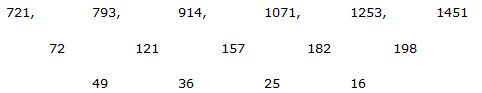The difference of difference is, 72, 62, 52, 42,….

Series II pattern: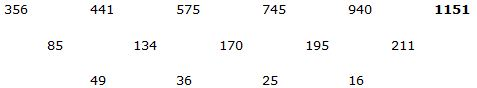The difference of difference is, 72, 62, 52, 42,….

Directions (6-10):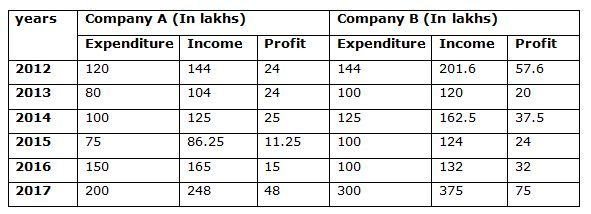Quantity I: In 2018, total income of company A and B is 500 lakhs and the expenditure of both companies is equal. Profit of company B is 20 lakhs more than the profit of company A and the profit percentage of company A is 20%. Company A’s profit is what percentage less than the company B’s profit?

Let us take income of company A in the year 2018 be x lakhs

Income of company B in the year 2018 be (x+20) lakhs

Total income of company A and B in the year 2018 = 500 lakhs

X+x+20= 500

2x = 500 – 20 => 480

= > x = 240 lakhs

Income of company A in the year 2018 = 120% of Expenditure of company A in the year 2018

240 = 120/100 * Expenditure of company A in the year 2018

Expenditure of company A in the year 2018 = (240/120)*100 = 200 lakhs

Company A’s profit = 240 – 200 = 40 lakhs

Company B’s income = 260 lakhs

Company B’s expenditure = 260 – (40+20)

= 260 – 60 = 200 lakhs

Required percentage = [(60 – 40)/60] * 100

= > (20/60) * 100 = 33 (1/3) %

Quantity II: Total income of company A and B in the year 2014 is approximately what percentage more/less than the total income of company A and B together in the year 2016?

Total income of company A and B in the year 2014 = (125 + 162.5) = 287.5 lakhs

Total income of company A and B in the year 2016 = (165 + 132) = 297 lakhs

Required percentage = [(297 – 287.5)/297] * 100 = 3.19 %

Quantity III: Total expenditure of company B in the year 2013 and 2014 together is what percentage more/less than the total expenditure of company A in the year 2012 and 2014 together?

Total expenditure of company B in the year 2012 and 2014 = (100 +125)

= 225 lakhs

Total expenditure of company A in the year 2012 and 2014 = (120+100)

= 220 lakhs

Required percentage = [(225 – 220)/220] * 100

= 2.27%

Quantity I > Quantity II > Quantity III

Quantity I: Find the difference of total income of company A in the year 2015, 2016 and 2017 together to that of total expenditure of company B in the year 2012, 2013 and 2014 together?

Total income of company A in the year 2015, 2016 and 2017

= 75*(115/100) + 150*(110/100) + 200*(124/100)

= (86.25 + 165 + 248)

= 499.25 lakhs

Total expenditure of company B in the year 2012, 2013 and 2014

= (144 + 100 + 125)

= 369 lakhs

Required difference = 499.25 – 369 = 130.25 lakhs

Quantity II: What is the average income of company B in the year 2014 and 2015 together?

Total income of company B in the year 2014 and 2015 = (162.5 + 124) = 286.5

Required average = (286.5/2) = 143.25 lakhs

Quantity III: In 2012, total expenditure of company A is 20% more than the previous year. The income of company A in the year 2013 is 20% less than the income of company A in the year 2011. Find the profit of company A in the year 2011?

Expenditure of company A in the year 2012 = (120/100)*Expenditure of company B in the year 2011

120 = (120/100)*Expenditure of company A in the year 2011

Expenditure of company A in the year 2011 = (120/120)*100 = 100 lakhs

Income of company A in the year 2013 = (80/100)*Income of company A in the year 2011

104 = (80/100)*Income of company B in the year 2011

Income of company B in the year 2011 = (104/80)*100 = 130 lakhs

Profit of company B in the year 2011 = 130 – 100 = 30 lakhs

Quantity I < Quantity II > Quantity III

Quantity I: In 2015, if the expenditure of company A is decreased by 25% and the income of company A is increased by 30%. Find the new profit percentage of company A?

Expenditure of company A in the year 2015 after decrement = 75*(75/100)

= 56.25 lakhs

Income of company A in the year 2015 after increment = 86.25*(130/100)

= 112.125 lakhs

Required percentage = [(112.125 – 56.25)/56.25] * 100 = 99.33%

Quantity II: In 2016, if the income of company B is decreased by 20% and the profit is decreased by (x) %. Find the value of x, if the expenditure is same.

Income of company B in the year 2016 after decrement = 132 * 80/100

= 105.6 lakhs

Expenditure of company B in the year 2016 = 100 lakhs

Profit = 105.6 – 100 = 5.6 lakhs

Percentage of profit decreased = [(32 – 5.6)/32]*100 = 82.5 %

Quantity III: Total income of company B in the year 2013 is what percentage more/less than the total expenditure of company A in the same year?

Required percentage = [(120 – 80)/80]*100

= (40/80)*100 = 50%

Quantity I > Quantity II > quantity III

Quantity I: In Company A, expenditure in the year 2012, 2014 and 2016 is increased by x %, (x+10) % and (x+15) % respectively. Find the new income of company A in the year 2012, 2014 and 2016 together, if the total expenditure of company A in the year 2012, 2014 and 2016 together after increment is 439.5 lakhs and the profit percentage is same as previous?

Expenditure of company A in the year 2012, 2014 and 2016 after increment

= 439.5 lakhs

120 * (100+x)/100 + 100 * (100+x+10)/100 + 150 * (100+x+15)/100 = 439.5

120 * (100+x) + 100 * (110+x)+150 * (115+x) = 43950

12000 + 120x + 11000 + 100x + 17250 + 150x = 43950

370x = 43950 – 17250 – 11000 – 12000

370x = 3700

= > x= 3700/370 = 10%

Expenditure of company A in the year 2012 after increment = 120 * 110/100

= 132 lakhs

Income of company A in the year 2012 after increment = 132 * 120/100

= 158.4 lakhs

Expenditure of company A in the year 2014 after increment = 100*120/100

= 120 lakhs

Income of company A in the year 2014 after increment = 120 * 125/100

= 150 lakhs

Expenditure of company A in the year 2016 after increment = 150 * 125/100

= 187.5 lakhs

Income of company A in the year 2016 after increment = 187.5 * 110/100

= 206.25 lakhs

Required total income of company A in the year 2012, 2014 and 2016

= (158.4 + 150 + 206.25) = 514.65 lakhs

Quantity II: In Company B, the income in the year 2013, 2015 and 2017 is decreased by 20%, 24% and 32% respectively. Find the new expenditure in the year 2013, 2015 and 2017 together if the profit percentage is same as previous?

Income of company B in the year 2013 after decrement = 120* 80/100

= 96 lakhs

Expenditure of company B in the year 2013 after decrement = 96*100/120

= 80 lakhs

Income of company B in the year 2015 after decrement = 124 * 76/100

= 94.24 lakhs

Expenditure of company B in the year 2015 after decrement = 94.24*100/124

= 76 lakhs

Income of company B in the year 2017 after decrement = 375 * 68/100

= 255 lakhs

Expenditure of company B in the year 2017 after decrement = 255 * 100/125

= 204 lakhs

Required total = 80 + 76 + 204 = 360 lakhs

Quantity III: Find the total income of company B in the year 2014, 2015 and 2016 together?

Required total income = 162.5 + 124 + 132 = 418.5 lakhs

Quantity I > Quantity II < Quantity III

Quantity I: Find the sum of the third highest and lowest income of company A in the given years?

Required sum = 144 + 86.25 = 230.25 lakhs

Quantity II: Find the sum of the second highest and second lowest expenditure of company B in the given years?

Required sum =144 + 100 = 244 lakhs

Quantity III: Find the sum of the highest and second highest profit of company A in the given years?

Required sum = 48 + 25 = 73 lakhs

Quantity I < Quantity III > Quantity II